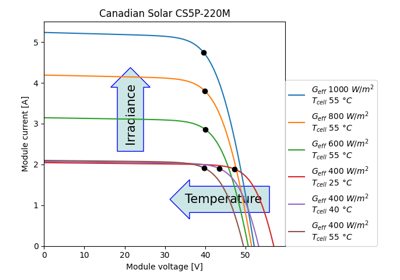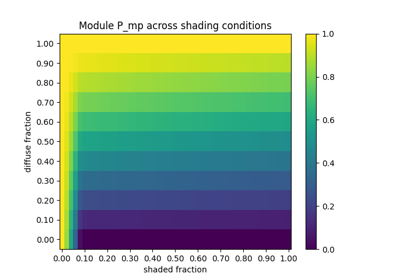# pvlib.pvsystem.calcparams_desoto#

pvlib.pvsystem.calcparams_desoto(effective_irradiance, temp_cell, alpha_sc, a_ref, I_L_ref, I_o_ref, R_sh_ref, R_s, EgRef=1.121, dEgdT=- 0.0002677, irrad_ref=1000, temp_ref=25)[source]#

Calculates five parameter values for the single diode equation at effective irradiance and cell temperature using the De Soto et al. model described in 1. The five values returned by calcparams_desoto can be used by singlediode to calculate an IV curve.

Parameters
• effective_irradiance (numeric) – The irradiance (W/m2) that is converted to photocurrent.

• temp_cell (numeric) – The average cell temperature of cells within a module in C.

• alpha_sc (float) – The short-circuit current temperature coefficient of the module in units of A/C.

• a_ref (float) – The product of the usual diode ideality factor (n, unitless), number of cells in series (Ns), and cell thermal voltage at reference conditions, in units of V.

• I_L_ref (float) – The light-generated current (or photocurrent) at reference conditions, in amperes.

• I_o_ref (float) – The dark or diode reverse saturation current at reference conditions, in amperes.

• R_sh_ref (float) – The shunt resistance at reference conditions, in ohms.

• R_s (float) – The series resistance at reference conditions, in ohms.

• EgRef (float) – The energy bandgap at reference temperature in units of eV. 1.121 eV for crystalline silicon. EgRef must be >0. For parameters from the SAM CEC module database, EgRef=1.121 is implicit for all cell types in the parameter estimation algorithm used by NREL.

• dEgdT (float) – The temperature dependence of the energy bandgap at reference conditions in units of 1/K. May be either a scalar value (e.g. -0.0002677 as in 1) or a DataFrame (this may be useful if dEgdT is a modeled as a function of temperature). For parameters from the SAM CEC module database, dEgdT=-0.0002677 is implicit for all cell types in the parameter estimation algorithm used by NREL.

• temp_ref (float (optional, default=25)) – Reference cell temperature in C.

Returns

• Tuple of the following results

• photocurrent (numeric) – Light-generated current in amperes

• saturation_current (numeric) – Diode saturation curent in amperes

• resistance_series (float) – Series resistance in ohms

• resistance_shunt (numeric) – Shunt resistance in ohms

• nNsVth (numeric) – The product of the usual diode ideality factor (n, unitless), number of cells in series (Ns), and cell thermal voltage at specified effective irradiance and cell temperature.

References

1(1,2)

W. De Soto et al., “Improvement and validation of a model for photovoltaic array performance”, Solar Energy, vol 80, pp. 78-88, 2006.

2

System Advisor Model web page. https://sam.nrel.gov.

3

A. Dobos, “An Improved Coefficient Calculator for the California Energy Commission 6 Parameter Photovoltaic Module Model”, Journal of Solar Energy Engineering, vol 134, 2012.

4

O. Madelung, “Semiconductors: Data Handbook, 3rd ed.” ISBN 3-540-40488-0

Notes

If the reference parameters in the ModuleParameters struct are read from a database or library of parameters (e.g. System Advisor Model), it is important to use the same EgRef and dEgdT values that were used to generate the reference parameters, regardless of the actual bandgap characteristics of the semiconductor. For example, in the case of the System Advisor Model library, created as described in , EgRef and dEgdT for all modules were 1.121 and -0.0002677, respectively.

This table of reference bandgap energies (EgRef), bandgap energy temperature dependence (dEgdT), and “typical” airmass response (M) is provided purely as reference to those who may generate their own reference module parameters (a_ref, IL_ref, I0_ref, etc.) based upon the various PV semiconductors. Again, we stress the importance of using identical EgRef and dEgdT when generation reference parameters and modifying the reference parameters (for irradiance, temperature, and airmass) per DeSoto’s equations.

Crystalline Silicon (Si):
• EgRef = 1.121

• dEgdT = -0.0002677

```>>> M = np.polyval([-1.26E-4, 2.816E-3, -0.024459, 0.086257, 0.9181],
...                AMa)
```

Source: 

• EgRef = 1.475

• dEgdT = -0.0003

```>>> M = np.polyval([-2.46E-5, 9.607E-4, -0.0134, 0.0716, 0.9196],
...                AMa)
```

Source: 

Copper Indium diSelenide (CIS):
• EgRef = 1.010

• dEgdT = -0.00011

```>>> M = np.polyval([-3.74E-5, 0.00125, -0.01462, 0.0718, 0.9210],
...                AMa)
```

Source: 

Copper Indium Gallium diSelenide (CIGS):
• EgRef = 1.15

• dEgdT = ????

```>>> M = np.polyval([-9.07E-5, 0.0022, -0.0202, 0.0652, 0.9417],
...                AMa)
```

Source: Wikipedia

Gallium Arsenide (GaAs):
• EgRef = 1.424

• dEgdT = -0.000433

• M = unknown

Source: 

## Examples using `pvlib.pvsystem.calcparams_desoto`#Calculating a module’s IV curves

Calculating a module's IV curvesCalculating power loss from partial module shading

Calculating power loss from partial module shading